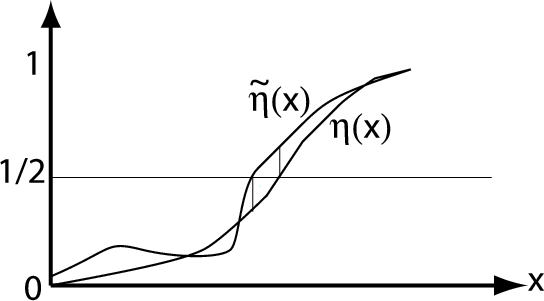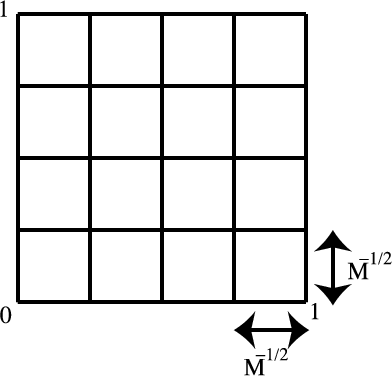0.5 Plug-in classifier and histogram classifier

 Page 1 / 2

We return to the topic of classification, and we assume an input (feature) space $\mathcal{X}$ and a binary output (label) space $\mathcal{Y}=\left\{0,1\right\}$ . Recall that the Bayes classifier (which minimizes the probability of misclassification) is defined by

${f}^{*}\left(x\right)=\left\{\begin{array}{cc}1,\phantom{\rule{0.277778em}{0ex}}\phantom{\rule{0.277778em}{0ex}}\hfill & P\left(Y=1|X=x\right)\ge 1/2\hfill \\ 0,\phantom{\rule{0.277778em}{0ex}}\phantom{\rule{0.277778em}{0ex}}\hfill & otherwise\hfill \end{array}\right).$

Throughout this section, we will denote the conditional probability function by

$\begin{array}{ccc}\hfill \eta \left(x\right)& \equiv & P\left(Y=1|X=x\right)\hfill \end{array}.$

Plug-in classifiers

One way to construct a classifier using the training data ${\left\{{X}_{i},{Y}_{i}\right\}}_{\phantom{\rule{4pt}{0ex}}i=1}^{n}$ is to estimate $\eta \left(x\right)$ and then plug-it into the form of the Bayes classifier. That is obtain an estimate,

${\stackrel{^}{\eta }}_{n}\left(x\right)=\eta \left(x;{\left\{{X}_{i},{Y}_{i}\right\}}_{\phantom{\rule{4pt}{0ex}}i=1}^{n}\right)$

and then form the “plug-in" classification rule

$\stackrel{^}{f}\left(x\right)=\left\{\begin{array}{cc}1,\phantom{\rule{0.277778em}{0ex}}\phantom{\rule{0.277778em}{0ex}}\hfill & \stackrel{^}{\eta }\left(x\right)\ge 1/2\hfill \\ 0,\phantom{\rule{0.277778em}{0ex}}\phantom{\rule{0.277778em}{0ex}}\hfill & otherwise\hfill \end{array}\right).$
The function $\eta \left(x\right)$ is generally more complicated than the ultimate classification rule (binary-valued), as we cansee
$\begin{array}{ccc}\hfill \eta & :& \mathcal{X}\to \left[0,1\right]\hfill \\ \hfill f& :& \mathcal{X}\to \left\{0,1\right\}\hfill \end{array}.$

Therefore, in this sense plug-in methods are solving a more complicated problem than necessary. However, plug-in methods can perform well,as demonstrated by the next result.

Theorem

Plug-in classifier

Let $\stackrel{˜}{\eta }$ be an approximation to $\eta$ , and consider the plug-in rule

$f\left(x\right)=\left\{\begin{array}{cc}1,\phantom{\rule{0.277778em}{0ex}}\phantom{\rule{0.277778em}{0ex}}\hfill & \stackrel{˜}{\eta }\left(x\right)\ge 1/2\hfill \\ 0,\phantom{\rule{0.277778em}{0ex}}\phantom{\rule{0.277778em}{0ex}}\hfill & otherwise\hfill \end{array}\right).$

Then,

$R\left(f\right)-{R}^{*}\le 2E\left[|\eta \left(x\right)-\stackrel{˜}{\eta }\left(x\right)|\right]$

where

$\begin{array}{ccc}\hfill R\left(f\right)& =& P\left(f\left(X\right)\ne Y\right)\hfill \\ \hfill {R}^{*}& =& R\left({f}^{*}\right)=\underset{f}{inf}R\left(f\right)\hfill \end{array}.$

Consider any $x\in {\mathbf{R}}^{d}$ . In proving the optimality of the Bayes classifier ${f}^{*}$ in Lecture 2 , we showed that

$\begin{array}{c}\hfill P\left(f,\left(,x,\right),\ne ,Y,|,X,=,x\right)-P\left({f}^{*},\left(x\right)\ne Y|X=x\right)\phantom{\rule{4pt}{0ex}}=\phantom{\rule{4pt}{0ex}}\left(2,\eta ,\left(,x,\right),-,1\right)\left[{\mathbf{1}}_{\left\{{f}^{*}\left(x\right)=1\right\}},-,{\mathbf{1}}_{\left\{f\left(x\right)=1\right\}}\right],\end{array}$

which is equivalent to

$\begin{array}{c}\hfill P\left(f,\left(,x,\right),\ne ,Y,|,X,=,x\right)-P\left({f}^{*},\left(x\right)\ne Y|X=x\right)\phantom{\rule{4pt}{0ex}}=\phantom{\rule{4pt}{0ex}}\left|2,\eta ,\left(,x,\right),-,1\right|\phantom{\rule{0.166667em}{0ex}}{\mathbf{1}}_{\left\{{f}^{*}\left(x\right)\ne f\left(x\right)\right\}},\end{array}$

since ${f}^{*}\left(x\right)=1$ whenever $2\eta \left(x\right)-1>0$ . Thus,

$\begin{array}{ccc}\hfill P\left(f\left(X\right)\ne Y\right)-{R}^{*}& =& {\int }_{{\mathbf{R}}^{d}}2|\eta \left(x\right)-1/2|\phantom{\rule{0.166667em}{0ex}}{\mathbf{1}}_{\left\{{f}^{*}\left(x\right)\ne f\left(x\right)\right\}}{p}_{X}\left(x\right)dx\hfill \\ & & \text{where}\phantom{\rule{4.pt}{0ex}}{p}_{X}\left(x\right)\phantom{\rule{4.pt}{0ex}}\text{is}\phantom{\rule{4.pt}{0ex}}\text{the}\phantom{\rule{4.pt}{0ex}}\text{marginal}\phantom{\rule{4.pt}{0ex}}\text{density}\phantom{\rule{4.pt}{0ex}}\text{of}\phantom{\rule{4.pt}{0ex}}X\hfill \\ & \le & {\int }_{{\mathbf{R}}^{d}}2|\eta \left(x\right)-\stackrel{˜}{\eta }\left(x\right)|{\mathbf{1}}_{\left\{{f}^{*}\left(x\right)\ne f\left(x\right)\right\}}{p}_{X}\left(x\right)dx\hfill \\ & \le & {\int }_{{\mathbf{R}}^{d}}2|\eta \left(x\right)-\stackrel{˜}{\eta }\left(x\right)|{p}_{X}\left(x\right)dx\hfill \\ & =& 2E\left[|\eta \left(X\right)-\stackrel{˜}{\eta }\left(X\right)|\right]\hfill \end{array}$

where the first inequality follows from the fact

$\begin{array}{ccc}\hfill f\left(x\right)\ne {f}^{*}\left(x\right)& ⇒& |\eta \left(x\right)-\stackrel{˜}{\eta }\left(x\right)|\ge |\eta \left(x\right)-1/2|\hfill \end{array}$

and the second inequality is simply a result of the fact that ${\mathbf{1}}_{\left\{{f}^{*}\left(x\right)\ne f\left(x\right)\right\}}$ is either 0 or 1.Pictorial illustration of | η ( x ) - η ˜ ( x ) | ≥ | η ( x ) - 1 / 2 | when f ( x ) ≠ f * ( x ) . Note that the inequality P ( f ( X ) ≠ Y ) - R * ≤ ∫ R d 2 | η ( x ) - η ˜ ( x ) | 1 { f * ( x ) ≠ f ( x ) } p X ( x ) d x shows that the excess risk is at most twice the integral over the setwhere f * ( x ) ≠ f ( x ) . The difference | η ( x ) - η ˜ ( x ) | may be arbitrarily large away from this set without effecting the error rate of the classifier. Thisillustrates the fact that estimating η well everywhere (i.e., regression) is unnecessary for the design of a good classifier (weonly need to determine where η crosses the 1 / 2 -level). In other words, “classification is easier than regression.”

The theorem shows us that a good estimate of $\eta$ can produce a good plug-in classification rule. By “good" estimate, we mean an estimator $\stackrel{˜}{\eta }$ that is close to $\eta$ in expected ${L}_{1}\text{-norm}$ .

The histogram classifier

Let's assume that the (input) features are randomly distributed over theunit hypercube $\mathcal{X}={\left[0,1\right]}^{d}$ (note that by scaling and shifting any set of bounded features we can satisfy this assumption),and assume that the (output) labels are binary, i.e., $\mathcal{Y}=\left\{0,1\right\}$ . A histogram classifier is based on a partition the hypercube ${\left[0,1\right]}^{d}$ into $M$ smaller cubes of equal size.

Partition of hypercube in 2 dimensions

Consider the unit square ${\left[0,1\right]}^{2}$ and partition it into $M$ subsquares of equal area (assuming $M$ is a squared integer). Let the subsquares be denoted by $\left\{{Q}_{i}\right\},\phantom{\rule{4pt}{0ex}}i=1,...,M$ .Example of hypercube [ 0 , 1 ] 2 in M equally sized partition

Define the following piecewise-constant estimator of $\eta \left(x\right)$ :

${\stackrel{^}{\eta }}_{n}\left(x\right)=\sum _{j=1}^{M}{\stackrel{^}{P}}_{j}{\mathbf{1}}_{\left\{x\in {Q}_{j}\right\}}$

where

${\stackrel{^}{P}}_{j}=\frac{{\sum }_{i=1}^{n}{\mathbf{1}}_{\left\{{X}_{i}\in {Q}_{j},{Y}_{i}=1\right\}}}{{\sum }_{i=1}^{n}{\mathbf{1}}_{\left\{{X}_{i}\in {Q}_{j}\right\}}}.$

Like our previous denoising examples, we expect that the bias of ${\stackrel{^}{\eta }}_{n}$ will decrease as $M$ increases, but the variance will increase as $M$ increases.

Is there any normative that regulates the use of silver nanoparticles?
what king of growth are you checking .?
Renato
What fields keep nano created devices from performing or assimulating ? Magnetic fields ? Are do they assimilate ?
why we need to study biomolecules, molecular biology in nanotechnology?
?
Kyle
yes I'm doing my masters in nanotechnology, we are being studying all these domains as well..
why?
what school?
Kyle
biomolecules are e building blocks of every organics and inorganic materials.
Joe
anyone know any internet site where one can find nanotechnology papers?
research.net
kanaga
sciencedirect big data base
Ernesto
Introduction about quantum dots in nanotechnology
what does nano mean?
nano basically means 10^(-9). nanometer is a unit to measure length.
Bharti
do you think it's worthwhile in the long term to study the effects and possibilities of nanotechnology on viral treatment?
absolutely yes
Daniel
how to know photocatalytic properties of tio2 nanoparticles...what to do now
it is a goid question and i want to know the answer as well
Maciej
Abigail
for teaching engĺish at school how nano technology help us
Anassong
Do somebody tell me a best nano engineering book for beginners?
there is no specific books for beginners but there is book called principle of nanotechnology
NANO
what is fullerene does it is used to make bukky balls
are you nano engineer ?
s.
fullerene is a bucky ball aka Carbon 60 molecule. It was name by the architect Fuller. He design the geodesic dome. it resembles a soccer ball.
Tarell
what is the actual application of fullerenes nowadays?
Damian
That is a great question Damian. best way to answer that question is to Google it. there are hundreds of applications for buck minister fullerenes, from medical to aerospace. you can also find plenty of research papers that will give you great detail on the potential applications of fullerenes.
Tarell
what is the Synthesis, properties,and applications of carbon nano chemistry
Mostly, they use nano carbon for electronics and for materials to be strengthened.
Virgil
is Bucky paper clear?
CYNTHIA
carbon nanotubes has various application in fuel cells membrane, current research on cancer drug,and in electronics MEMS and NEMS etc
NANO
so some one know about replacing silicon atom with phosphorous in semiconductors device?
Yeah, it is a pain to say the least. You basically have to heat the substarte up to around 1000 degrees celcius then pass phosphene gas over top of it, which is explosive and toxic by the way, under very low pressure.
Harper
Do you know which machine is used to that process?
s.
how to fabricate graphene ink ?
for screen printed electrodes ?
SUYASH
What is lattice structure?
of graphene you mean?
Ebrahim
or in general
Ebrahim
in general
s.
Graphene has a hexagonal structure
tahir
On having this app for quite a bit time, Haven't realised there's a chat room in it.
Cied
what is biological synthesis of nanoparticles
how did you get the value of 2000N.What calculations are needed to arrive at it
Privacy Information Security Software Version 1.1a
Good
Got questions? Join the online conversation and get instant answers!By Prateek AshtikarByBy